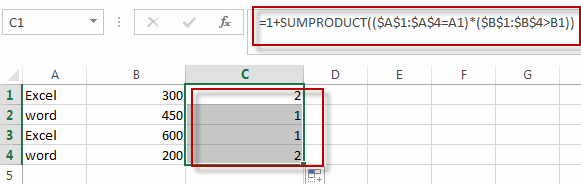# Rank Data with Multiple Criteria

This post will guide you how to ranking with multiple criteria in excel. How do I rank data with multiple criteria in excel. How to rank data with multiple conditions in excel. How to rank data with multiple references using an excel formula.

## Rank Data with Multiple Criteria

If you want to rank data with only one condition or one reference, you just only need to use the RANK function to easily rank values in a range of cells in excel. Assuming that you want to rank data against multiple criteria for a range of cells in your worksheet. How to achieve it. You need to create a new complex formula based on the SUMPRODUCT function. For example, you want to rank a list of data in the range of cells A1:B4 with two references or criteria, just using the following formula:

`=1+SUMPRODUCT((\$A\$1:\$A\$4=A1)*(\$B\$1:\$B\$4>B1))`

You need to type this formula into the formula box of cell C1, and then press Enter key, and drag the AutoFill Handler over other cells.

Let’s see the result:### Related Functions

• Excel SUMPRODUCT function
The Excel SUMPRODUCT function multiplies corresponding components in the given one or more arrays or ranges, and returns the sum of those products.The syntax of the SUMPRODUCT function is as below:= SUMPRODUCT (array1,[array2],…)…